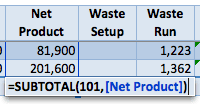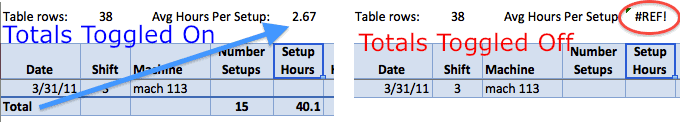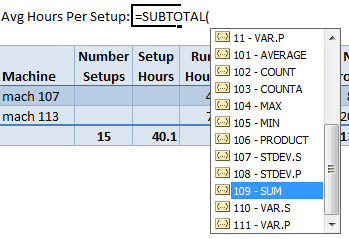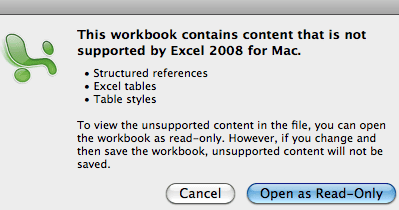# Total Row Formulas in Excel Tables

Excel Tables have a Total Row that can be toggled on and off from the Ribbon. Several functions are available when the Total Row is turned on.

In Excel 2010 and 2007 (Windows) select a cell inside the Table, then choose Table Tools and click the check box for Total Row. In Excel 2011 (Mac) select a cell in the Table and choose Tables, then select the check box for Total Row.A Total row appears below the data where each column has access to several automatic formulas. The default selection for the Total Row is none, meaning no function is selected when you first turn on the Total Row on your Table.Average, Count, Count Numbers, Max, Min, Sum, StdDev, and Var are options on the drop-down list for each cell in the Total Row. You can even choose More Functions…, which we won’t go into here.

When you choose a function from the list, what you really get is the SUBTOTAL function, where the first argument is the function, and the second argument is the Table column data. In the picture below, 101 represents the Average function, and [Net Product] is the data. Excel inserts this formula automatically when you select Average from the drop-down list.Using SUBTOTAL in the Total Row means that when the Table is Filtered, the function will give the correct answer.

If you toggle the Total Row off, Excel remembers the function and it will appear again when you toggle the Total Row back on.

## Total Row Reference in a Formula

The Total Row can be used in a formula outside the Table. Notice the Avg Hours Per Setup formula below uses #Totals to refer to the Total Row in the Table.By using the the Setup Hours and Number Setups from the Total Row, you can Filter the Table and get the correct answer for Average Hours per Setup.

The problem occurs when the Total Row is toggled off, which leaves this formula without a reference.If you recall from my last post on Tables, I used the following formula for Average Hours Per Setup =SUM(Table1[Setup Hours])/SUM(Table1[Number Setups]) which has a different flaw. It does not work when the Table is Filtered.

## Create a Formula That Works

We can replace SUM in this last formula with the SUBTOTAL function =SUBTOTAL(109,Table1[Setup Hours])/SUBTOTAL(109,Table1[Number Setups]).Now when we Filter the Table the calculation is correct and we don’t have to worry about having the Total Row toggled on for the formula to work.

## SUBTOTAL with Windows and Mac

It’s much easier to use the SUBTOTAL Function with Windows versions of Excel (2007, 2010) because autocomplete gives you the different function numbers for the first argument.The Mac version (2011) doesn’t have autocomplete but you can access Help, which brings you to another window with some information that helps you decide which function number to use for SUBTOTAL.Hidden values refers to entire rows that are hidden. Excel Tables use “Ignores hidden values” as function numbers when you select a function from the drop-down list in the Total Row.

## Table Availability in Excel Versions

As you may have guessed  Excel 2003 (Windows) doesn’t have Tables. They have what is referred to as Lists, which is the pre-cursor to Tables. Lists don’t have any structured data.

The same is true for Excel 2008 (Mac), which only has Lists and no Tables. If you have a file with Tables and open it with an earlier version having only Lists then you get a warning.The moral of this is to upgrade, Tables are worth it.